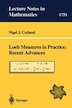Loeb Measures In Practice: Recent Advances: EMS Lectures 1997

byNigel J. Cutland

Pricing and Purchase Info

\$59.23 online
\$64.95 list price save 8%
Earn 296 plum® points

Prices and offers may vary in store

Quantity:

In stock online

Ships free on orders over \$25

Not available in stores

This expanded version of the 1997 European Mathematical Society Lectures given by the author in Helsinki, begins with a self-contained introduction to nonstandard analysis (NSA) and the construction of Loeb Measures, which are rich measures discovered in 1975 by Peter Loeb, using techniques from NSA. Subsequent chapters sketch a range of recent applications of Loeb measures due to the author and his collaborators, in such diverse fields as (stochastic) fluid mechanics, stochastic calculus of variations ("Malliavin" calculus) and the mathematical finance theory. The exposition is designed for a general audience, and no previous knowledge of either NSA or the various fields of applications is assumed.
Title:Loeb Measures In Practice: Recent Advances: EMS Lectures 1997Format:PaperbackDimensions:250 pagesPublished:December 12, 2000Publisher:Springer Berlin HeidelbergLanguage:English

The following ISBNs are associated with this title:

ISBN - 10:3540413847

ISBN - 13:9783540413844

Reviews

1. Loeb Measures
1.1 Introduction
1.2 Nonstandard Analysis
1.2.1 The hyperreals
1.2.2 The nonstandard universe
1.2.3 N1-saturation
1.2.4 Nonstandard topology
1.3 Construction of Loeb Measures
1.3.1 Example: Lebesgue measure
1.3.2 Example: Haar measure
1.3.3 Example: Wiener measure
1.3.4 Loeb measurable functions 1.4 Loeb Integration Theory
1.5 Elementary Applications
1.5.1 Lebesgue integration
1.5.2 Peano's Existence Theorem
1.5.3 Ito integration and stochastic differential equations 2 Stochastic Fluid Mechanics
2.1 Introduction
2.1.1 Function spaces
2.1.2 Functional formulation of the Navier-Stokes equations
2.1.3 Definition of solutions to the stochastic Navier-Stokes equations
2.1.4 Nonstandard topology in Hilbert spaces
2.2 Solution of the Deterministic Navier-Stokes Equations
2.2.1 Uniqueness
2.3 Solution of the Stochastic Navier-Stokes Equations
2.3.1 Stochastic Flow
2.3.2 Nonhomogeneous stochastic Navier-Stokes equations
2.4 Stochastic Euler Equations
2.5 Statistical Solutions
2.5.1 The Foias equation
2.5.2 Construction of statistical solutions using Loeb measures
2.5.3 Measures by nonstandard densities
2.5.4 Construction of statistical solutions using nonstandard densities
2.5.5 Statistical solutions for stochastic Navier-Stokes equations
2.6 Attractors for the Navier-Stokes Equations
2.6.1 Introduction
2.6.2 Nonstandard attractors and standard attractors
2.6.3 Attractors for 3-dimensional Navier-Stokes equations
2.7 Measure Attractors for Stochastic Navier-Stokes Equations
2.8 Stochastic Attractors for Navier-Stokes Equations
2.8.1 Stochastic attractors
2.8.2 Existence of a stochastic attractor for the Navier-Stokes equations
2.9 Attractors for the 3-dimensional Stochastic Navier-Stokes Equations
3. Stochastic Calculus of Variations
3.1 Introduction
3.1.1 Notation
3.2 Flat Integral Representation of Wiener Measure
3.3 The Wiener Sphere
3.4 Brownian Motion on the Wiener Sphere and the Infinite Dimensional Ornstein-Uhlenbeck Process
3.5 Malliavin Calculus
3.5.1 Notation and preliminaries
3.5.2 The Wiener-Ito chaos decomposition
3.5.3 The derivation operator
3.5.4 The Skorohod integral
3.5.5 The Malliavin operator
4. Mathematical Finance Theory
4.1 Introduction
4.2 The Cox-Ross-Rubinstein Models
4.3 Options and Contingent Claims
4.3.1 Pricing a claim
4.4 The Black-Scholes Model
4.5 The Black-Scholes Model and Hyperfinite CRR Models
4.5.1 The Black-Scholes formula
4.5.2 General claims
4.6 Convergence of Market Models
4.7 Discretisation Schemes
4.8 Further Developments
4.8.1 Poisson pricing models
4.8.2 American options 4.8.3 Incomplete markets
4.8.4 Fractional Brownian motion
4.8.5 Interest rates
Index